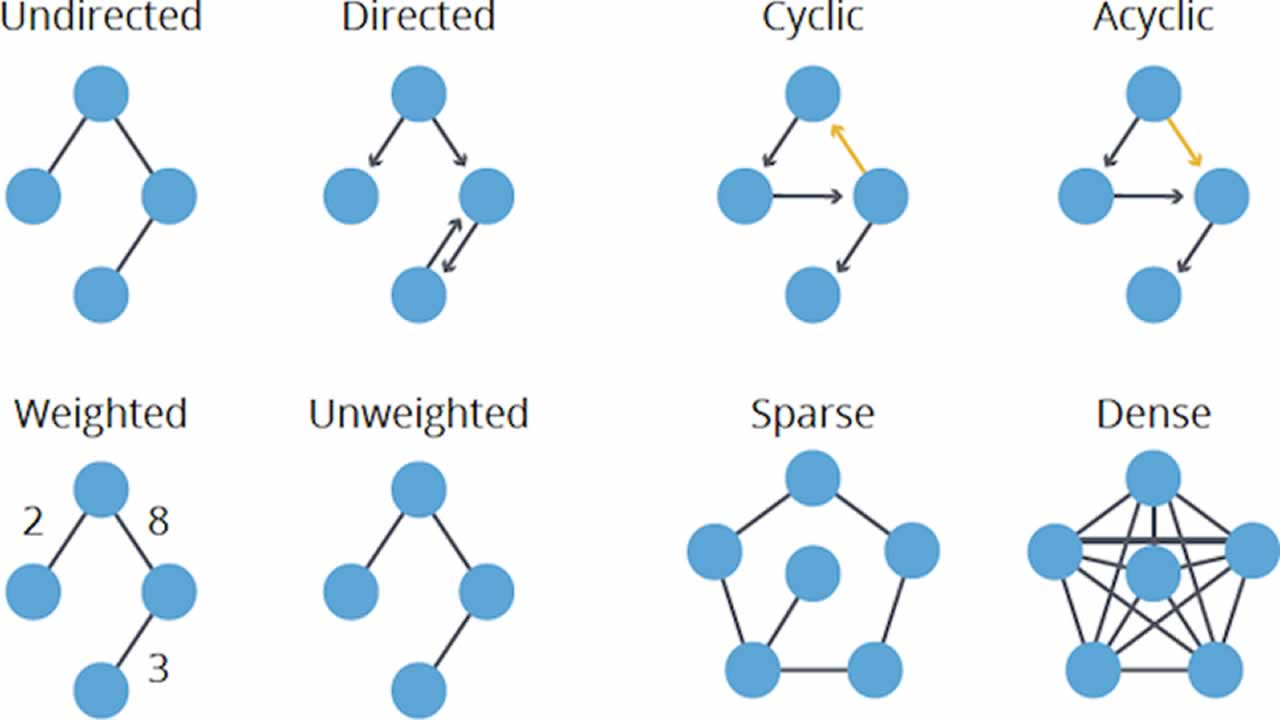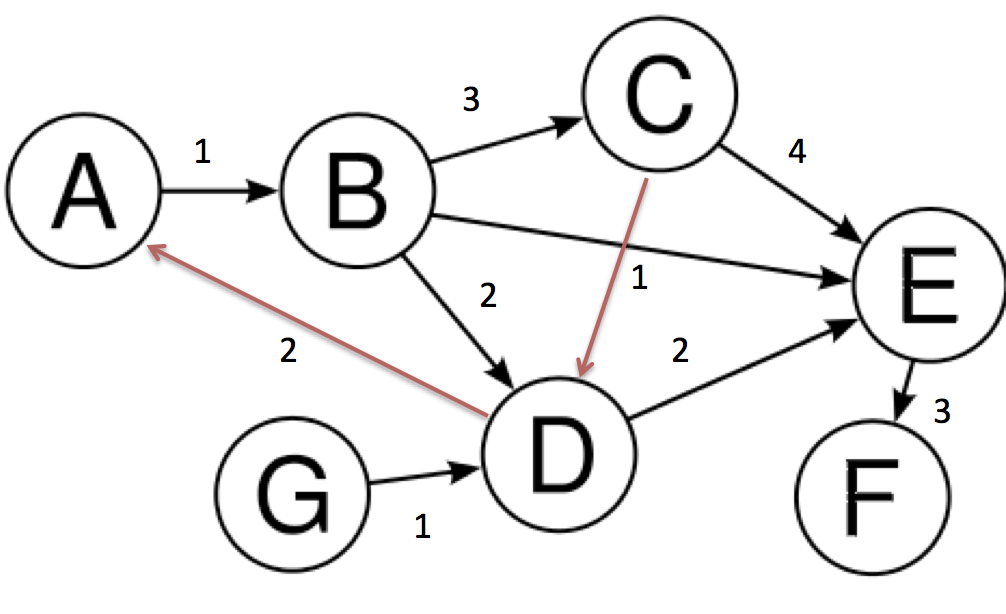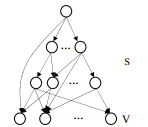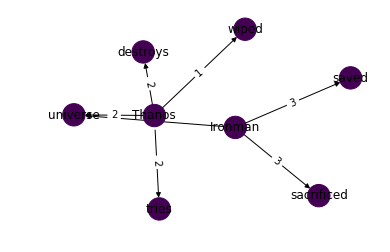×

Search anything:

# Pachinko Allocation Model (PAM)

#### Machine Learning (ML)Get this book -> Problems on Array: For Interviews and Competitive Programming

Reading time: 30 minutes | Coding time: 15 minutes

Pachinko Allocation Model (PAM) is a topic modeling technique which is an improvement over the shortcomings of Latent Dirichlet Allocation. In this article at OpenGenus, we have explained it in detail.

## Need for a better model

We have covered popular topic modeling techniques like Latent Dirichlet Allocation, Latent Semantic Index, Non-Negative Matrix Factorization etc. All of these models are very powerful in obtaining the semantic relationships between words present in the documents. But they donot show any coherent relationship between the topics generated. So we need even more powerful models to achieve that. It is highly required for text summarization techniques. Especially in case of Abstraction based text summarization methods, this feature would be highly necessary. Currently, there are many news apps which implements this technique which uses abstraction based text summarization methods for generating the summary of the news.

Semantic relationships are the associations that exists between meanings of words or pharses or sentences. Coherent relationships are marked by an orderly, logically, gramatically and aesthetically consistent relation of words in sentences.

## Here come the Saviour

Inability to extract the relationship between topics poses a huge limitation of LDA method. Because in a continuous passage, the succeeding line would have certain coherence with its preceeding line. So it is highly important to obtain tight coherence between passages to obtain proper topics. This difficulty is overcome by using Pachinko Allocation Model.

It captures arbitrary, nested and even sparse correlation between topics using Directed Acyclic Graph. The list of all words obtained from the corpus after removing the stopwords and text processing represents the dirichlet distribution. In a PAM, each of the topic generated is associated with the dirichlet distribution through a Directed Acyclic Graph (DAG).

## Directed Acyclic Graph

A Directed Acyclic Graph is a non cyclic finite directed graphs. The below diagrams explains the types of graphing networks and the functionality of DAG.It consists of mainly two components node and link.

01. Node
The topics that are to be connected together are called nodes. Therefore, each node represents a topic. Attributes of the topic represents the words that are related to the particular topic.

The links represents the connections between the nodes. In our model the links are acyclic and are directed. Thus arises the name.

## Functioning

In this model, each leaf node corresponds to the words present in the vocabulary and each non-leaf node represents the topics. In an arbitrary DAG, a LDA model would not have the links between those non leaf interior nodes whereas the PAM model uses the links between them to have a tight complete coherence throughout the corpus.

The model is named after pachinko machines - a popular game in Japan in which a metal ball bounces downwards across a complex set of pins and obstacles until they reach various bins at the bottom.Let us now look at the architecture of PAM. The following image explains the structural arrangement of PAM model.In the above picture the top node represents the root node. The second level hierarchy represents the super topics. the third level hierarchy represents the sub topics. Finally, the bottom level represents the vocabulary.

## Working

For example, let us consider the following sentences.

Thanos wiped out half of the universe. Thanos then tries to destroy the whole universe. Ironman sacrificed his life to save the whole universe.

In the above passage, the topics of first sentence can be Thanos, wiped, universe. The topics of second sentence can be Thanos,tries, destroy, universe. The topics of third sentence can be Ironman, sacrificed, save, universe.

Here when we use regular LDA methods, it can clearly pick out good topics from the passage. But when we look at the relationship between topics, it cannot help. But a PAM can maintain relationships between multiple topics. In the first two sentences, we have Thanos in common. In all the three sentences, we have universe in common. Thus, PAM helps in obtaining the summary of the text.

Therefore in the above passage, the individual words form the vocabulary, the topics that I have listed out represents the sub topics. The coherent words like Thanos and universe represents the super topics.

Now let us implement this in python. Let us first import all the required packages to start working.

``````import networkx as nx
import numpy as np
import matplotlib.pyplot as plt
import pylab
``````

In particular to these above mentioned sentences, let us add the topics to see it visually.

``````G = nx.DiGraph()

``````

Nodes are also represented as vertices and links are also known as connections or edges. Now let us generate the visual representation of PAM.

``````values = [val_map.get(node, 0.45) for node in G.nodes()]
edge_labels=dict([((u,v,),d['weight'])
for u,v,d in G.edges(data=True)])

edge_colors = ['black'  for edge in G.edges()]

pos=nx.spring_layout(G)
nx.draw_networkx_edge_labels(G,pos,edge_labels=edge_labels, with_labels=True)
nx.draw(G,pos, node_color = values, node_size=500,edge_color=edge_colors,edge_cmap=plt.cm.Reds, with_labels=True)
pylab.show()
``````

The output of the program is the following image showing the relationship between the individual topics.The graph is created based on the frequency, choerent relationships between words. The topics, subtopics are extracted by this model itself. This model doesnot have its implementation in popular libraries such as scikit learn or keras since it is a relatively new method. But it is available in a python library called tomatopy. It can be accessed through tomotopy.PAModel code. But it is not widely used. An example implementation of this model is given below.

``````import tomotopy as tp
mdl = tp.PAM(k1=5, k2=25)
for docs in corpus:
mdl.train(1000)

# first, infer the document
doc_inst = mdl.make_doc(docs_to_be_inferred)
mdl.infer(doc_inst)

# next, count subtopics
subtopic_counts =  * mdl.k2
for t in doc_inst.subtopics:
subtopic_counts[t] += 1

# estimate distribution
total = sum(subtopic_counts)
for i in range(mdl.k2):
print('Subtopic #{} : {}'.format(i, subtopic_counts[i] / tot))
``````

## Alternative methods

There are various methods and tools available for showing the relationship between coherent words. Some of the tools are:

1. Neo4j
2. SAP HANA
3. Mongo DB

These are noSQL databases to display potential information from databases other than relational database.

## Applications

1. These approaches are highly useful in text summarization techniques especially in abstractive text summarization where the actually passage is paraphrased into a short passage.

2. It posses high value in marketing analytics which is used in obtaining the potential regions and potential set of customers and trying to improve their sales there. It can also be used to find out the regions with comparatively less sales and concentrate on their growth in that region.

3. It is also used in operations to find out the optimal method of transportation and also finding out the best possible location to expand their business or plant based on the PAM on social network data.